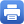Columbia Home
Complex Analysis and Riemann Surfaces

This course is taken in sequence, part 1 in the fall, and part 2 in the spring.

### Complex Analysis and Riemann Surfaces I

I Holomorphic Functions

• Holomorphic functions, Cauchy-Riemann equations
• Conformal mappings
• Cauchy integral formula, residues

II Analytic Continuation

• Gamma and zeta functions
• Hypergeometric functions and monodromy
• Braid group representations
• Correlation functions in conformal field theory

III Riemann Surfaces

• The Riemann surface y2=x(x-1)(x-l)
• Holomorphic and meromorphic differentials
• Homology, fundamental group, surface classification
• Weierstrass elliptic functions
• Theta functions
• The moduli space of tori
• Introduction to Riemann surfaces of arbitrary genera
• Fields of meromorphic functions, field extensions, Galois theory

### Complex Analysis and Riemann Surfaces II

I Theta Functions and Modular Forms

• Modular transformations and modular forms
• Eisenstein series, Dedekind eta-function, Kronecker limit formula
• Hecke operators
• Poisson summation, theta-functions of lattices
• Exact formulas for heat kernels

II Selected Topics, chosen from

• Integrable models, spectral curves, and solitons
• Modular forms and infinite-dimensional algebras
• Geometry of the moduli space of Riemann surfaces
• Solvable models in statistical mechanics or conformal field theory
• Introduction to L-functionsPrint this page